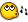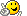# my first gui calc

## Recommended Posts

this is my first scripttry not

to rip meapart to bad

um... always rememberthat you have

to compile itfirst in order for it to work

calculater.au3

division.au3

multiplacation.au3

subtraction.au3

##### Share on other sites

this is my first scripttry not

to rip meapart to bad

um... always rememberthat you have

to compile itfirst in order for it to work

<{POST_SNAPBACK}>

ok... can some one at least try my script and/or tell me whats wrong with it

"crickets"##### Share on other sites

ok... can some one at least try my script and/or tell me whats wrong with it

"crickets"<{POST_SNAPBACK}>

just copy and paste it all but just put it on new pages on scite like addition subtraction ecetera

and reply how it is##### Share on other sites

```#region --- GuiBuilder code Start ---
; Script generated by AutoBuilder 0.5 Prototype

#include <GuiConstants.au3>

\$Button_3 = GuiCtrlCreateButton("_-~calculate ~-_", 110, 140, 110, 40)
\$Button_4 = GuiCtrlCreateButton("<----back", 10, 10, 80, 20)
GuiSetState()
While 1
\$msg = GuiGetMsg()
Select
Case \$msg = \$GUI_EVENT_CLOSE
MsgBox(0, "are you sure?", "are you sure you would like to exit? click ok below")
\$1 = InputBox("exit?", "type yes to exit or if not just close this box")
if \$1 = "yes" then ExitLoop
Case \$msg = \$button_1
if @error then ContinueLoop
GUICtrlSetData(\$button_1,\$input)
Case \$msg = \$button_2
if @error then ContinueLoop
GUICtrlSetData(\$button_2,\$input)
Case \$msg = \$button_3
\$messege = MsgBox(0,"", \$input + \$iinput)
if @error then ContinueLoop
Case \$msg = \$button_4
Run("calculater.exe")
ExitLoop
if @error then ContinueLoop
EndSelect
WEnd
Exit
#endregion --- GuiBuilder generated code End ---```
##### Share on other sites

subtraction

```#region --- GuiBuilder code Start ---
; Script generated by AutoBuilder 0.5 Prototype

#include <GuiConstants.au3>
GuiCreate("subtraction", 392, 322,(@DesktopWidth-392)/2, (@DesktopHeight-322)/2 )

\$Button_3 = GuiCtrlCreateButton("_-~calculate ~-_", 110, 140, 110, 40)
\$Button_4 = GuiCtrlCreateButton("<----back", 10, 10, 80, 20)
GuiSetState()
While 1
\$msg = GuiGetMsg()
Select
Case \$msg = \$GUI_EVENT_CLOSE
MsgBox(0, "are you sure?", "are you sure you would like to exit? click ok below")
\$1 = InputBox("exit?", "type yes to exit or if not just close this box")
if \$1 = "yes" then ExitLoop
Case \$msg = \$button_1
\$input = InputBox("subtracted", "type 1 number below to be subtracted")
if @error then ContinueLoop
GUICtrlSetData(\$button_1,\$input)
Case \$msg = \$button_2
\$iinput = InputBox("subtracted", "type 1 number below to be subtracted")
if @error then ContinueLoop
GUICtrlSetData(\$button_2,\$input)
Case \$msg = \$button_3
\$messege = MsgBox(0,"", \$input - \$iinput)
if @error then ContinueLoop
Case \$msg = \$button_4
Run("calculater.exe")
ExitLoop
if @error then ContinueLoop
EndSelect
WEnd
Exit
#endregion --- GuiBuilder generated code End ---```
##### Share on other sites

multiplacation

```#region --- GuiBuilder code Start ---
; Script generated by AutoBuilder 0.5 Prototype

#include <GuiConstants.au3>
GuiCreate("multiplacation", 392, 322,(@DesktopWidth-392)/2, (@DesktopHeight-322)/2 )

\$Button_3 = GuiCtrlCreateButton("_-~calculate ~-_", 110, 140, 110, 40)
\$Button_4 = GuiCtrlCreateButton("<----back", 10, 10, 80, 20)
GuiSetState()
While 1
\$msg = GuiGetMsg()
Select
Case \$msg = \$GUI_EVENT_CLOSE
MsgBox(0, "are you sure?", "are you sure you would like to exit? click ok below")
\$1 = InputBox("exit?", "type yes to exit or if not just close this box")
if \$1 = "yes" then ExitLoop
Case \$msg = \$button_1
\$input = InputBox("multiplacation", "type 1 number below to be multiplied")
if @error then ContinueLoop
GUICtrlSetData(\$button_1,\$input)
Case \$msg = \$button_2
\$iinput = InputBox("multiplacation", "type 1 number below to be multiplied")
if @error then ContinueLoop
GUICtrlSetData(\$button_2,\$input)
Case \$msg = \$button_3
\$messege = MsgBox(0,"", \$input * \$iinput)
if @error then ContinueLoop
Case \$msg = \$button_4
Run("calculater.exe")
ExitLoop
if @error then ContinueLoop

;;;
EndSelect
WEnd
Exit
#endregion --- GuiBuilder generated code End ---```
##### Share on other sites

division

```#region --- GuiBuilder code Start ---
; Script generated by AutoBuilder 0.5 Prototype

#include <GuiConstants.au3>
GuiCreate("division", 392, 322,(@DesktopWidth-392)/2, (@DesktopHeight-322)/2 )

\$Button_3 = GuiCtrlCreateButton("_-~calculate ~-_", 110, 140, 110, 40)
\$Button_4 = GuiCtrlCreateButton("<----back", 10, 10, 80, 20)
GuiSetState()
While 1
\$msg = GuiGetMsg()
Select
Case \$msg = \$GUI_EVENT_CLOSE
MsgBox(0, "are you sure?", "are you sure you would like to exit? click ok below")
\$1 = InputBox("exit?", "type yes to exit or if not just close this box")
if \$1 = "yes" then ExitLoop
Case \$msg = \$button_1
\$input = InputBox("division", "type 1 number below to be divided")
if @error then ContinueLoop
GUICtrlSetData(\$button_1,\$input)
Case \$msg = \$button_2
\$iinput = InputBox("division", "type 1 number below to be divided")
if @error then ContinueLoop
GUICtrlSetData(\$button_2,\$input)
Case \$msg = \$button_3
\$messege = MsgBox(0,"", \$input / \$iinput)
if @error then ContinueLoop
Case \$msg = \$button_4
Run("calculater.exe")
ExitLoop
if @error then ContinueLoop
EndSelect
WEnd
Exit
#endregion --- GuiBuilder generated code End ---```
##### Share on other sites

calculater

```#region --- GuiBuilder code Start ---
; Script generated by AutoBuilder 0.5 Prototype

#include <GuiConstants.au3>
GuiCreate("CalCUlaTEr", 392, 322,(@DesktopWidth-392)/2, (@DesktopHeight-322)/2)

\$Button_1 = GuiCtrlCreateButton("multiplacation", 100, 40, 170, 20)
\$Button_2 = GuiCtrlCreateButton("division", 100, 70, 170, 20)
\$Button_3 = GuiCtrlCreateButton("addition", 100, 100, 170, 20)
\$Button_4 = GuiCtrlCreateButton("subtraction", 100, 130, 170, 20)

GuiSetState()
While 1
\$msg = GuiGetMsg()
Select
Case \$msg = \$GUI_EVENT_CLOSE
MsgBox(0, "are you sure?", "are you sure you would like to exit? click ok below")
\$1 = InputBox("exit?", "type yes to exit or if not just close this box")
if \$1 = "yes" then ExitLoop
Case \$msg = \$button_1
run("multiplacation.exe")
ExitLoop
if @error then ContinueLoop
Case \$msg = \$button_2
Run("division.exe")
ExitLoop
if @error then ContinueLoop
Case \$msg = \$button_3
ExitLoop
if @error then ContinueLoop
Case \$msg = \$button_4
Run("subtraction.exe")
ExitLoop
if @error then ContinueLoop
EndSelect
WEnd
Exit
#endregion --- GuiBuilder generated code End ---```

## Create an account

Register a new account

• ### Recently Browsing   0 members

×

• Wiki

• Back

• #### Beta

• Git
• FAQ
• Our Picks
×
• Create New...